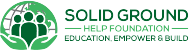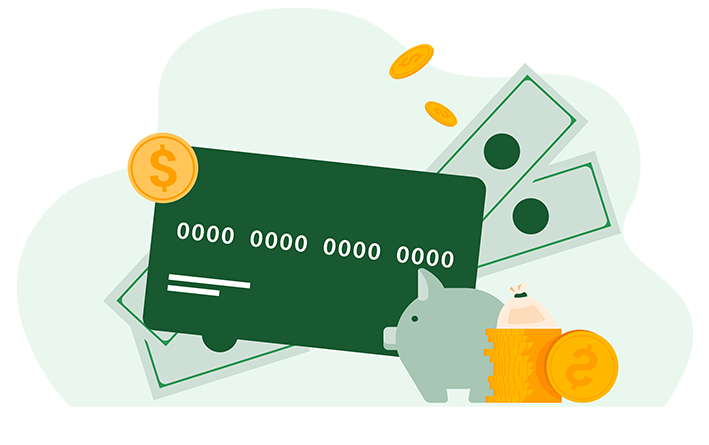Search## How Are Interest Rates Calculated

When you take out a loan, you`ll have to pay back both the amount you borrowed and interest on top of it. Usually, the repayments you make on a loan will be made up of two parts: the part that reduces your balance to pay off your loan (principal) and the part that covers the interest on the loan.Divide your interest rate by the number of payments you will make in the year (interest rates are expressed annually). So, for example, if you are making monthly payments, divide by 12. Next, multiply it by the balance of your loan, which for the first payment, will be your whole principal amount. So, for example, on a personal loan of \$30,000.00 at 8.40%, how much is the dollar amount you will pay off in 12 months? Also, how much will you pay for the entire year?

(Formula: Loan Amount (\$30,000.00 ) x Interest Rate (0.084) = \$2,520.00 annually. Next, you must divide by 12 months, which will give you the monthly amount of interest which equals 210 per month).

Divide your interest dollar amount, which in this example is \$2,520.00 by the number of payments you will make in the year (interest rates are expressed annually). So, for example, if you are making monthly payments, divide by 12. (\$2,520.00/12 = \$210).

Multiply it by the loan term. For example, 10 years, which is equivalent to 120 months \$210.00 x 120 months equals \$25,200.00, which is the amount that you will pay back in interest if you pay your loan in 10 years at 8.40%.Scroll to Top